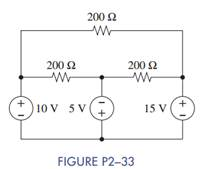Chat Now

# In Figure P2−33: (a) Assign a voltage and current variable to every element. (b) Use KVL to...

### In Figure P2−33: (a) Assign a voltage and current variable to every element. (b) Use KVL to...

In Figure P2−33:

(a) Assign a voltage and current variable to every element.

(b) Use KVL to find the voltage across each resistor.

(c) Use Ohm’s law to find the current through each resistor.

(d) Use KCL to find the current through each voltage source.manish jayant 28-Jul-2020Get solution